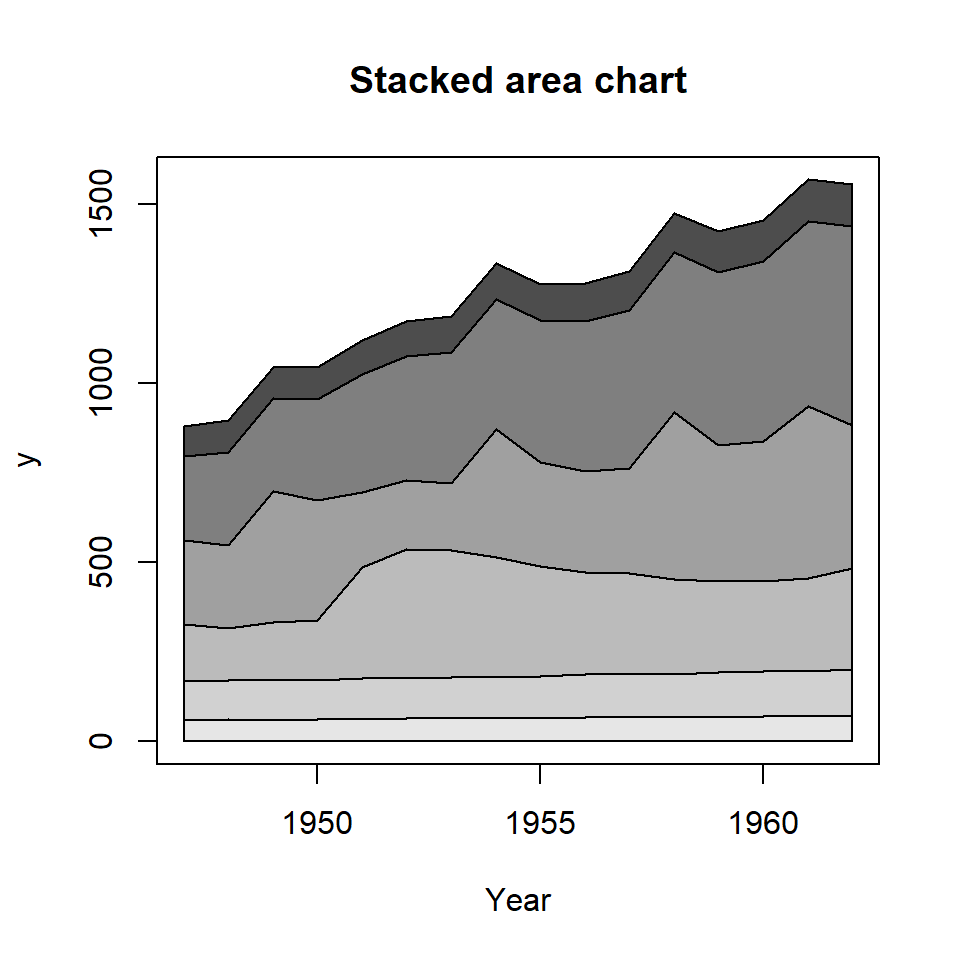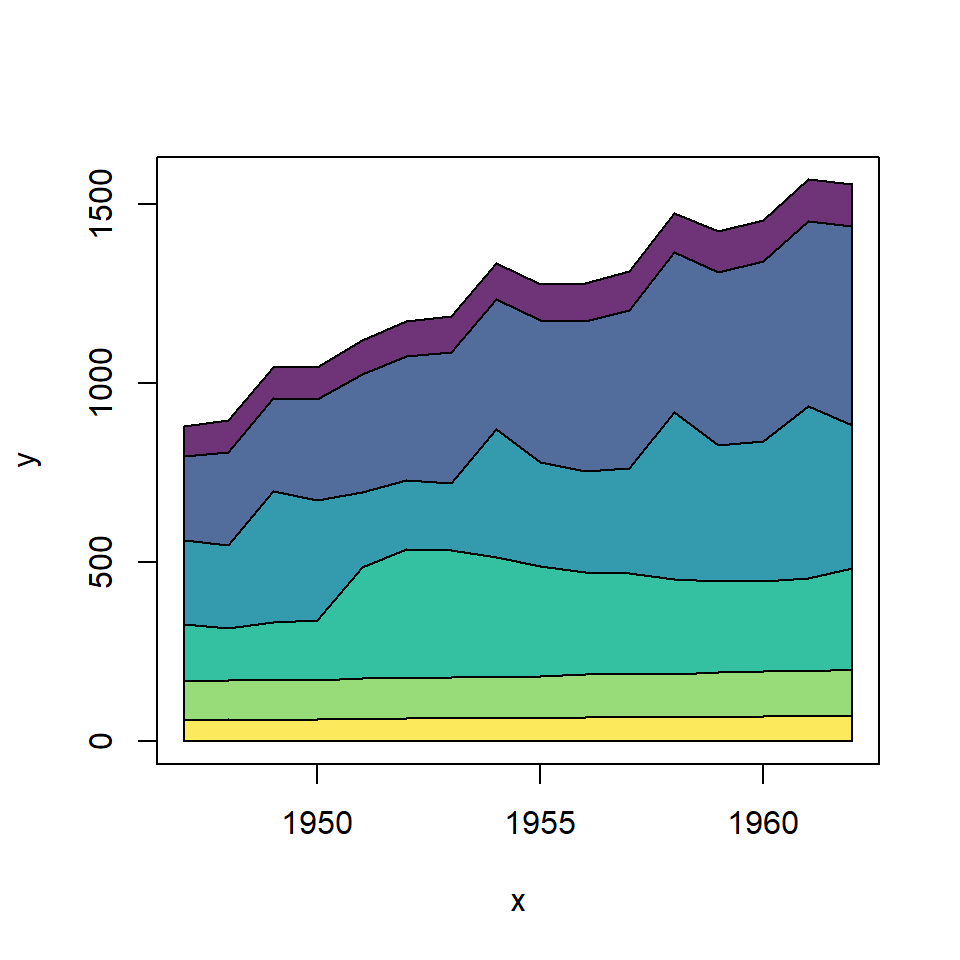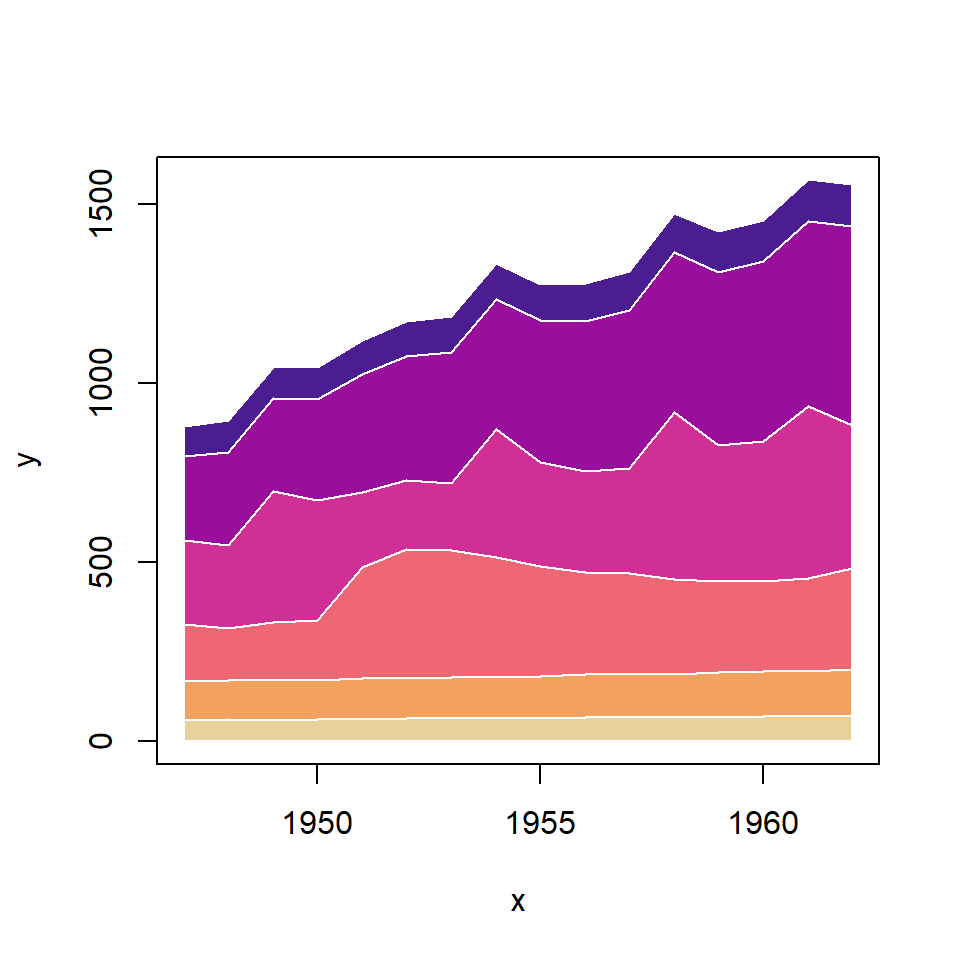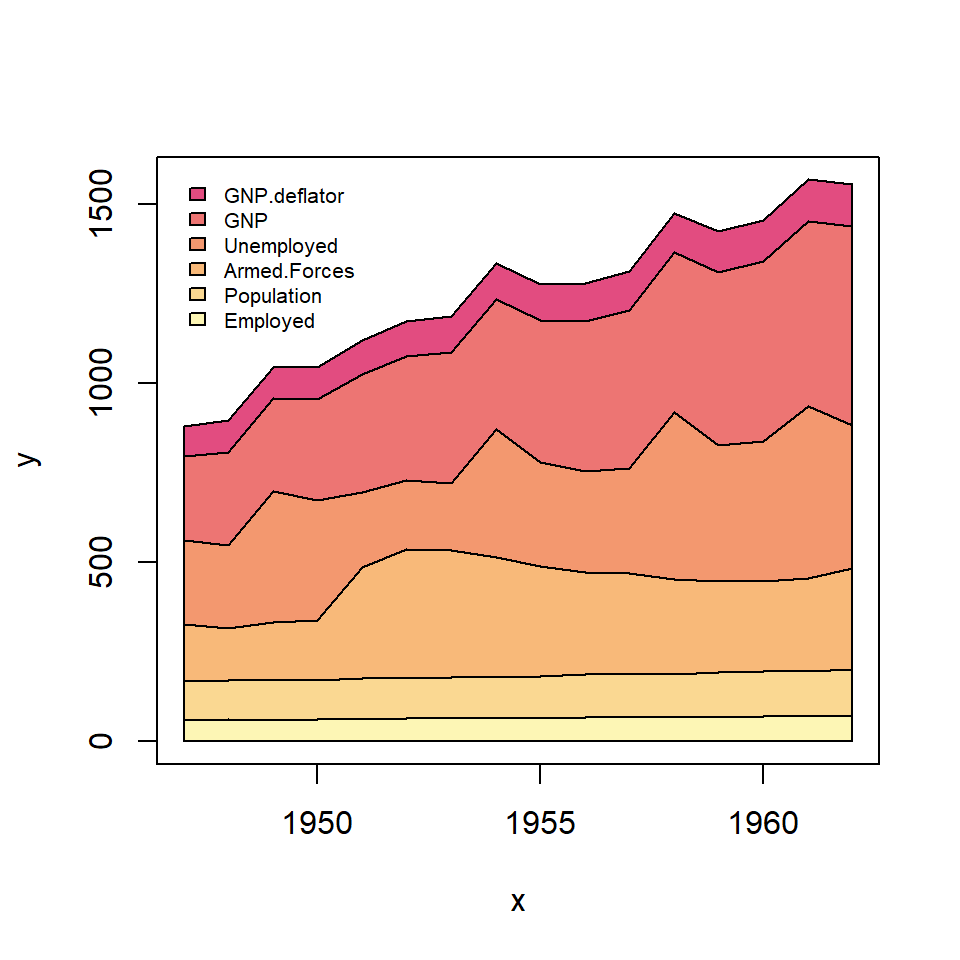# Stacked area plot in R

areaplot

Arni Magnusson

## Sample data set

Consider the `longley` data set, which contains macroeconomic data of several years.

``````# Sample dataset
df <- longley

# X-axis variable
x <- df\$Year

# Variables to be stacked
y <- df[, c(1, 2, 3, 4, 5, 7)]``````

## The `areaplot` function

The most straightforward way for creating a stacked area chart in base R is using the `areaplot` package, which contains a function of the same name for this purpose. Check also the percentange stacked area chart for a stacked chart where each serie is displayed as percentage.

You can pass two numerical variables to the function or specify a formula to create the area plot. Note that the default colors are in gray scale.

``````# install.packages("areaplot")
library(areaplot)

# Stacked area chart
areaplot(x, y,
main = "Stacked area chart",
xlab = "Year")

# Equivalent to:
areaplot(.~Year, data = df,
main = "Stacked area chart",
xlab = "Year")``````## Color customizationThe `cols` argument can be used to customize the color palette used to colorize each area. In the following example we are using the well-known viridis palette with 0.8 transparency.

``````# install.packages("areaplot")
library(areaplot)

# Colors
cols <- hcl.colors(6, palette = "viridis", alpha = 0.8)

# Stacked area chart with custom colors
areaplot(x, y,
col = cols)``````The lines style can be customized making use of additional arguments. For instance, you can customize the border color with `border` and change the line width and line type with `lwd` and `lty`, respectively.

``````# install.packages("areaplot")
library(areaplot)

# Colors
cols <- hcl.colors(6, palette = "ag_Sunset")

# Stacked area chart with custom borders
areaplot(x, y, col = cols,
border = "white",
lwd = 1,
lty = 1)``````

Setting `legend = TRUE` as an argument of the `areaplot` function a legend will be created automatically. You can override the default arguments for the legend with `args.legend`. See the legends article for more details.

``````# install.packages("areaplot")
library(areaplot)

# Colors
cols <- hcl.colors(6, palette = "PinkYl")

# Area plot with legend
areaplot(x, y, col = cols,
legend = TRUE,
args.legend = list(x = "topleft", cex = 0.65))``````